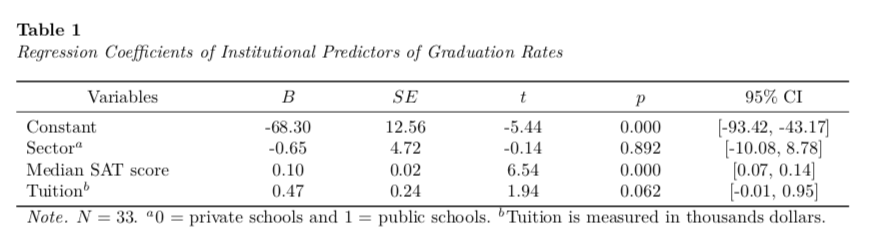# APA Tables using RMarkdown: Part 4

Markdown
R
Writing
Author

Andy

Published

January 13, 2020

This is the fourth part of a short blog series I am writing to re-create some of the sample tables found in the 7th edition APA Publication Manual. In this post I will attempt to mimic Table 7.17 (p. 220). To do so, I will incorporate many ideas that I covered in the first, second, and third of these posts.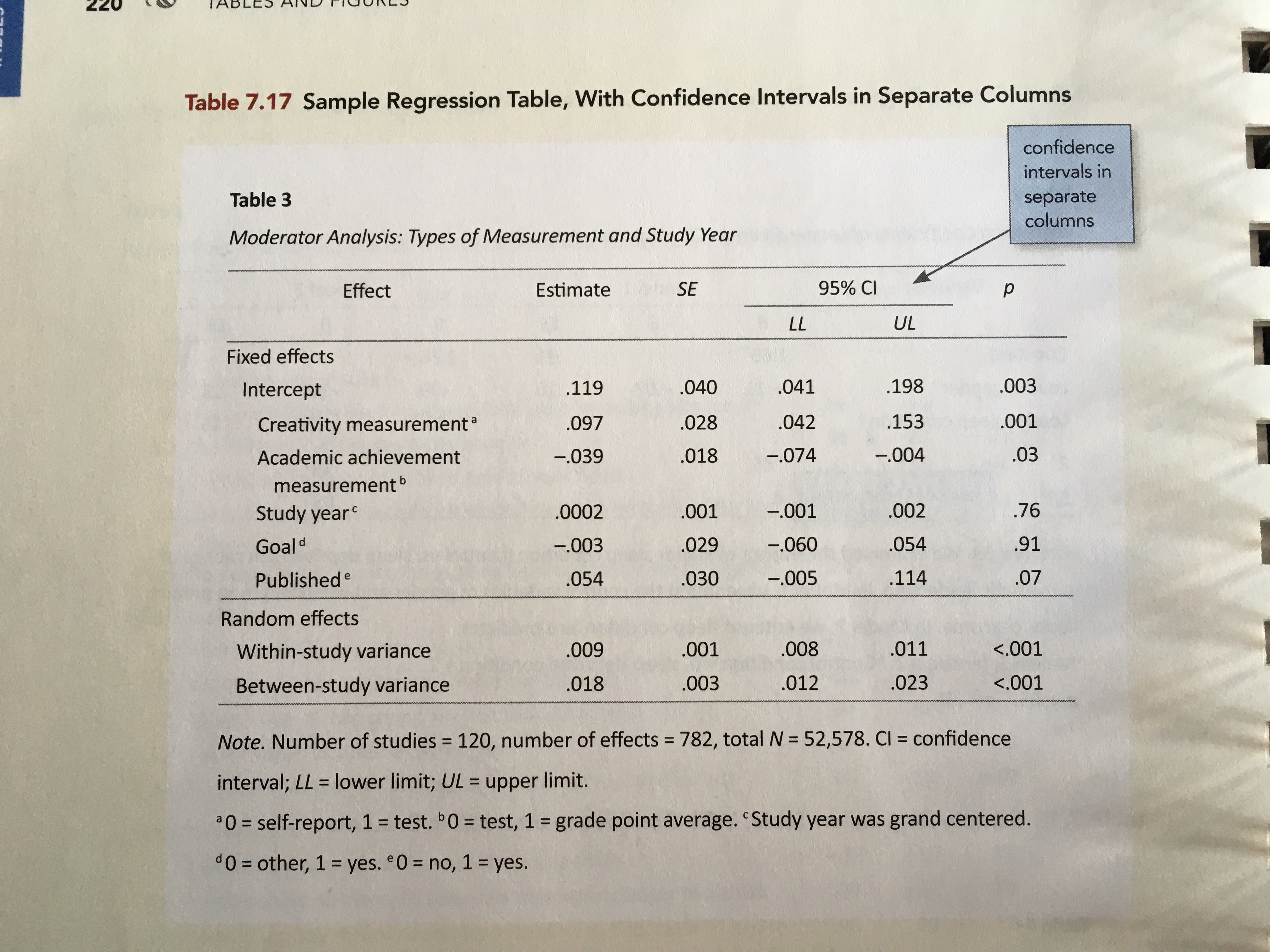Table 7.17 from the 7th edition of the APA Publication Manual (p. 220).

Rather than re-create this table using the data from Table 7.17, I will illustrate mimicing this table with summary information gleaned from a different dataset.

# My Process

I will again render to PDF and set up the YAML to import the caption package (LaTeX) and set up the APA caption formatting.

---
title: "Untitled"
author: "Andrew Zieffler"
date: "1/12/2020"
output: pdf_document
- \usepackage{caption}
- \captionsetup[table]{textfont={it}, labelfont={bf}, singlelinecheck=false, labelsep=newline}
---

In the RMarkdown body, I will load a few packages and also import a data set that I will use to create the summary values akin to those in Table 7.10.

# Load libraries
library(broom)
library(knitr)
library(kableExtra)
library(tidyverse)

# Import data
mutate(public = if_else(sector == "Public", 1, 0))

# View data
head(mn)
# A tibble: 6 × 6
name                               grad sector    sat tuition public
<chr>                             <dbl> <chr>   <dbl>   <dbl>  <dbl>
1 Augsburg College                   65.2 Private  1030    39.3      0
2 Bethany Lutheran College           52.6 Private  1065    30.5      0
3 Bethel University, Saint Paul, MN  73.3 Private  1145    39.4      0
4 Carleton College                   92.6 Private  1400    54.3      0
5 College of Saint Benedict          81.1 Private  1185    43.2      0
6 Concordia College at Moorhead      69.4 Private  1145    36.6      0

The data in were collected from http://www.collegeresults.org and contain 2011 institutional data for n=33 Minnesota colleges and universities. The codebook is available here.

We need to compute the regression results from fitting an OLS model. Here I will predict variation in graduation rates using the other variables in the dataset. I will also use the tidy() output from the broom package to obtain the coefficient-level output that is summarized in the table.

# Fit model
lm.1 = lm(grad ~ 1 + public + sat + tuition, data = mn)

# Obtain coefficient-level summaries
tab_01 = tidy(lm.1)

# View table
tab_01
# A tibble: 4 × 5
term        estimate std.error statistic     p.value
<chr>          <dbl>     <dbl>     <dbl>       <dbl>
1 (Intercept)  -68.3     12.6       -5.44  0.00000755
2 public        -0.647    4.72      -0.137 0.892
3 sat            0.104    0.0159     6.54  0.000000364
4 tuition        0.470    0.242      1.94  0.0617     

We also need to obtain the limits of the confidence intervals. We can simply take the estimate and add/subtract two standard errors to get these limits.

# Compute confidence limits
tab_01 = tab_01 %>%
mutate(
LL = estimate - 2*std.error,
UL = estimate + 2*std.error
)

# View table
tab_01
# A tibble: 4 × 7
term        estimate std.error statistic     p.value       LL      UL
<chr>          <dbl>     <dbl>     <dbl>       <dbl>    <dbl>   <dbl>
1 (Intercept)  -68.3     12.6       -5.44  0.00000755  -93.4    -43.2
2 public        -0.647    4.72      -0.137 0.892       -10.1      8.78
3 sat            0.104    0.0159     6.54  0.000000364   0.0721   0.136
4 tuition        0.470    0.242      1.94  0.0617       -0.0135   0.953

Now we just arrange the columns in the order we want to create the table and also re-name the elements in the term column to correspond to the text we want in the table.

# Select columns
tab_01 = tab_01 %>%
select(
term, estimate, std.error, LL, UL, p.value
) %>%
mutate(
term = c("Constant", "Sector$^a$", "Median SAT score", "Tuition$^b$")
)

# View table
tab_01
# A tibble: 4 × 6
term             estimate std.error       LL      UL     p.value
<chr>               <dbl>     <dbl>    <dbl>   <dbl>       <dbl>
1 Constant          -68.3     12.6    -93.4    -43.2   0.00000755
2 Sector$^a$         -0.647    4.72   -10.1      8.78  0.892
3 Median SAT score    0.104    0.0159   0.0721   0.136 0.000000364
4 Tuition$^b$         0.470    0.242   -0.0135   0.953 0.0617     

I will then pipe this into the kable() function to set the column names, column alignment, and table caption. The digits= argument is included to round the values in each column. (Since the first column is text we set this to NA.)

I also employ similar kableExtra function from those introduced in Part 1, Part 2, and Part 3 to make the table the full page width, include the footnote, center the header names, and increase the width of the first column, and add the top header row.

kable(
tab_01,
format = "latex",
booktabs = TRUE,
escape = FALSE,
col.names = c("", "", "", "$LL$", "$UL$", ""),
align = c("l", "c", "c", "c", "c", "c"),
digits = c(NA, 3, 3, 3, 3, 3),
caption = "Regression Coefficients of Institutional Predictors of Graduation Rates"
) %>%
kable_styling(full_width = TRUE) %>%
footnote(
general_title = "Note.",
general = "$N=33$. $^a$0 = private schools and 1 = public schools. $^b$Tuition is measured in thousands of dollars.",
footnote_as_chunk = TRUE,
escape = FALSE
) %>%
row_spec(row = 0, align = "c") %>%
column_spec(column = 1, width = "1.5in") %>%
c("Variable" = 1, "$B$" = 1, "$SE$" = 1, "95\\\\% CI" = 2, "$p$" = 1),
escape = FALSE
)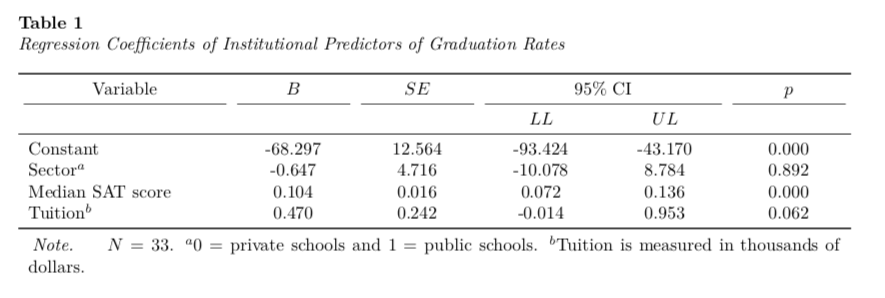We need to remove the extraneous mid-rules by altering the LaTeX syntax as we did in Part 2.

\begin{table}

\caption{\label{tab:}Regression Coefficients of Institutional Predictors of Graduation Rates}
\centering
\begin{tabu} to \linewidth {>{\raggedright\arraybackslash}p{1.5in}>{\centering}X>{\centering}X>{\centering}X>{\centering}X>{\centering}X}
\toprule
\multicolumn{1}{c}{Variable} & \multicolumn{1}{c}{$B$} & \multicolumn{1}{c}{$SE$} & \multicolumn{2}{c}{95\% CI} & \multicolumn{1}{c}{$p$} \\
\cmidrule(l{3pt}r{3pt}){4-5}
\multicolumn{1}{c}{} & \multicolumn{1}{c}{} & \multicolumn{1}{c}{} & \multicolumn{1}{c}{$LL$} & \multicolumn{1}{c}{$UL$} & \multicolumn{1}{c}{}\\
\midrule
Constant & -68.297 & 12.564 & -93.424 & -43.170 & 0.000\\
Sector$^a$ & -0.647 & 4.716 & -10.078 & 8.784 & 0.892\\
Median SAT score & 0.104 & 0.016 & 0.072 & 0.136 & 0.000\\
Tuition$^b$ & 0.470 & 0.242 & -0.014 & 0.953 & 0.062\\
\bottomrule
\multicolumn{6}{l}{\textit{Note.} $N=33$. $^a$0 = private schools and 1 = public schools. $^b$Tuition is measured in thousands of dollars.}\\
\end{tabu}
\end{table}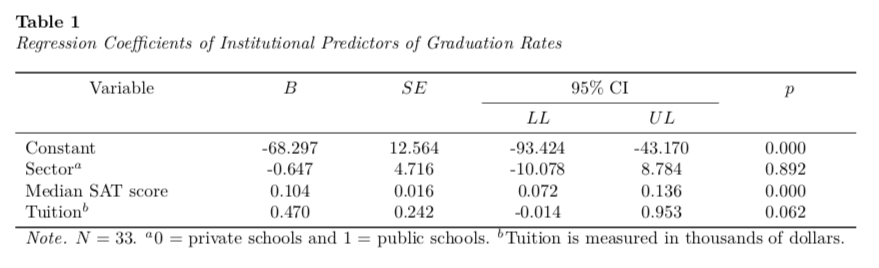# Alternative Table

An alternative table puts the confidence intevral in a single column by putting the confidence limits in square brackets. This is akin to Table 7.16 (p. 219) in the 7th edition of the APA Publication Manual.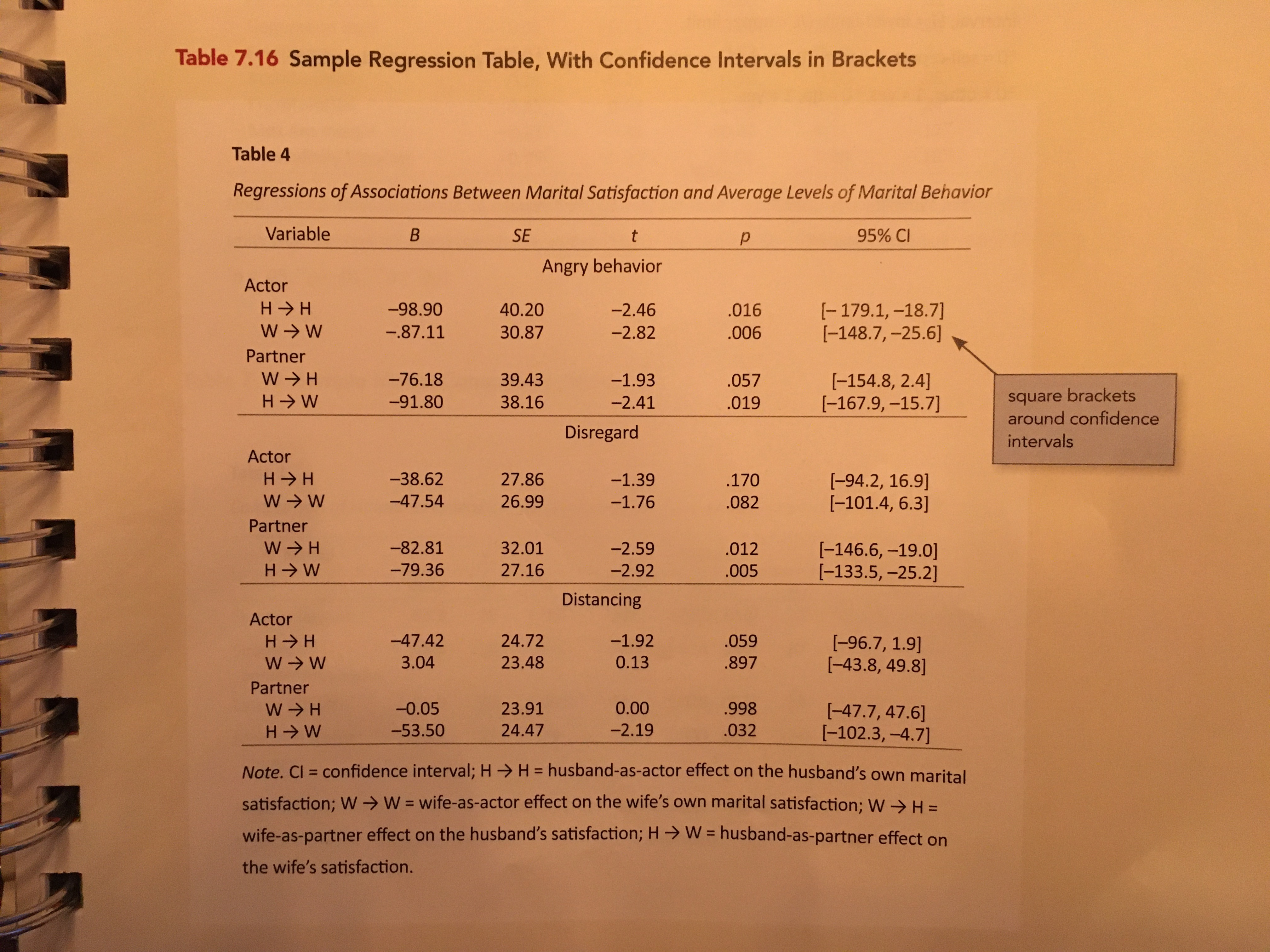Table 7.16 from the 7th edition of the APA Publication Manual (p. 219).

To create this I will again use the tidy() function and create the confidence limits. I will then create a new column in the tidy output that concatenates the confidence limits and places them inside square brackets. Lastly I will select the columns I want for the table and set the text for the “Variables” column.

# Get coefficient-level output
# Create confidence limits
tab_01 = tidy(lm.1) %>%
mutate(
LL = estimate - 2*std.error,
UL = estimate + 2*std.error
)

# Put confidence limits inside square brackets
tab_01$CI = paste0("[", round(tab_01$LL, 2), ", ", round(tab_01$UL, 2), "]") # Get output for table tab_01 = tab_01 %>% select( term, estimate, std.error, statistic, p.value, CI ) %>% mutate( term = c("Constant", "Sector$^a$", "Median SAT score", "Tuition$^b$") ) tab_01 # A tibble: 4 × 6 term estimate std.error statistic p.value CI <chr> <dbl> <dbl> <dbl> <dbl> <chr> 1 Constant -68.3 12.6 -5.44 0.00000755 [-93.42, -43.17] 2 Sector$^a$-0.647 4.72 -0.137 0.892 [-10.08, 8.78] 3 Median SAT score 0.104 0.0159 6.54 0.000000364 [0.07, 0.14] 4 Tuition$^b$0.470 0.242 1.94 0.0617 [-0.01, 0.95]  Finally, I will use kable() and the kableExtra functionality to create the table. kable( tab_01, format = "latex", booktabs = TRUE, escape = FALSE, col.names = c("Variables", "$B$", "$SE$", "$t$", "$p", "95\\% CI"), align = c("l", "c", "c", "c", "c", "c"), digits = c(NA, 2, 2, 2, 3, NA), caption = "Regression Coefficients of Institutional Predictors of Graduation Rates" ) %>% kable_styling(full_width = TRUE) %>% footnote( general_title = "Note.", general = "N=33$.$^a$0 = private schools and 1 = public schools.$^b\$Tuition is measured in thousands  dollars.",
footnote_as_chunk = TRUE,
escape = FALSE
) %>%
row_spec(row = 0, align = "c") %>%
column_spec(column = 1, width = "1.5in") %>%
column_spec(column = 6, width = "1in")